NCERT Solutions for Class 7 Maths Chapter 11 Perimeter and Area Ex 11.3 are part of NCERT Solutions for Class 7 Maths. Here we have given NCERT Solutions for Class 7 Maths Chapter 11 Perimeter and Area Ex 11.3.

 Board CBSE Textbook NCERT Class Class 7 Subject Maths Chapter Chapter 11 Chapter Name Perimeter and Area Exercise Ex 11.3 Number of Questions Solved 17 Category NCERT Solutions

## NCERT Solutions for Class 7 Maths Chapter 11 Perimeter and Area Ex 11.3

Question 1.
Find the circumference of the circles with the following radus (Take π = $$\frac { 22 }{ 7 }$$)
(a) 14 cm
(b) 28 mm
(c) 21 cm
Solution: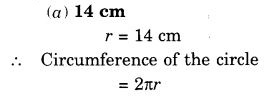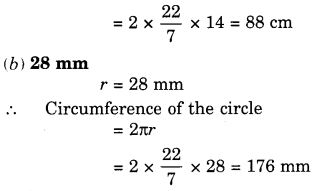Question 2.
Find the area of the following circles, given that: ( Take π = $$\frac { 22 }{ 7 }$$ )
(b) diameter = 49 m
Solution:Question 3.
If the circumference of a circular sheet is 154 m, find its radius. Also find the area of the sheet ( Take π = $$\frac { 22 }{ 7 }$$ )
Solution:
Circumference of the circular sheet = 154 m
Let the radius of the circular sheet be r cm
Then, its circumference = 2nr m According to the question,
Circumference = 2πr = 154Question 4.
A gardener wants to fence a circular garden of diameter 21 m. Find the length of the rope he needs to purchase if he makes 2 rounds of offense. Also find the cost of the rope, if it costs ₹ 4 per meter. ( Take π = $$\frac { 22 }{ 7 }$$ )
Solution:
The diameter of the circular garden (r) = 21 m
Radius of the circular garden (r) = $$\frac { 21 }{ 2 }$$ m
∴ Circumference of the circular garden = 2πr
= 2 × $$\frac { 22 }{ 7 }$$ × $$\frac { 21 }{ 2 }$$ m = 66m
⇒ Length of the rope needed to make 1 round of fence = 66 m
⇒ Length of the rope needed to make 2 rounds of fence
= 66 × 2 m = 132 m
Cost of rope per meter = ₹ 4
∴ Cost of the rope = ₹ 132 × 4 = ₹ 528.

Question 5.
From a circular sheet of radius 4 cm, a circle of radius 3 cm is removed. Find the area of the remaining sheet. (Take π = 3.14)
Solution: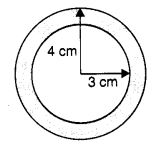Here, Outer radius, r = 4 cm
Inner radius, r = 3 cm
Area of the remaining sheet = Outer area – Inner area
= π (R2 – r2) = 3.14 (42 – 32) cm2
= 3.14 (16 – 9) cm2
= 3.14 × 7 cm2 = 21.98 cm2

Question 6.
Saima wants to put lace on the edge of a circular table cover of a diameter of 1.5 m. Find the length of the lace required and also find its cost if one meter of the lace costs ₹ 15. (Take π = 3.14)
Solution:
Diameter of the table cover = 1.5 m
⇒ Radius of the table cover (r) = $$\frac { 1.5 }{ 2 }$$ m
⇒ Circumference of the table cover = 2πr
= 2 × 3.14 × $$\frac { 1.5 }{ 2 }$$ m = 4.71 m
⇒ Length of the lace required = 4.71 m
∵ Cost of lace per meter = ₹ 15
∴ Cost of the lace = ₹ 4.71 × 15 = ₹ 70.65

Question 7.
Find the perimeter of the following figure, which is a semicircle including its diameter.
Solution:Question 8.
Find the cost of polishing a circular table-top of diameter 1.6 m, if the rate of polishing is ₹ 15/m2. (Take π = 3.14)
Solution:
Diameter of the table-top = 1.6 m
⇒ Radius of the table-top (r) = $$\frac { 1.6 }{ 2 }$$ m = 0.8 m
∴ Area of the table-top = πr2
= 3.14 × (0.8)2 m2
= 3.14 × 0.64 m2
= 2.0096 m2
∵ Rate of polishing = ₹ 15 per m2
∴ Cost of polishing the table-top = ₹ 2.0096 × 15
= ₹ 30.144
= ₹ 30.14 (approx.).

Question 9.
Shazli took a wire of length 44 cm and bent it into the shape of a circle. Find the radius of that circle. Also find its area. If the same wire is bent into the shape of a square, what will be the length of each of its side? Which figure encloses more area, the circle or the square? ( Take π = $$\frac { 22 }{ 7 }$$ )
Solution:Question 10.
From a circular card sheet of radius 14 cm, two circles of radius 3.5 cm and a rectangle of length 3 cm and breadth 1 cm are removed (as shown in the following figure). Find the area of the remaining sheet. ( Take π = $$\frac { 22 }{ 7 }$$ )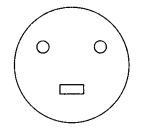Solution: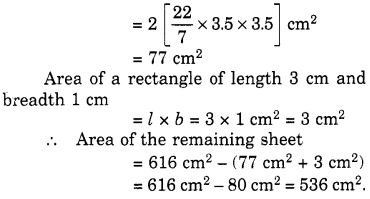Question 11.
A circle of radius 2 cm is cut out from a square piece of an aluminium sheet of side 6 cm. What is the area of the leftover aluminium sheet? (Take π = 3.14)
Solution:Question 12.
The circumference of a circle is 31.4 cm. Find the radius and the area of the circle? (Take π = 3.14)
Solution: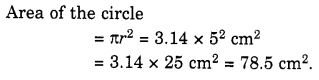Question 13.
A circular flower bed is surrounded by a path 4 m wide. The diameter of the flower bed is 66 m. What is the area of this path? (π = 3.14)Solution: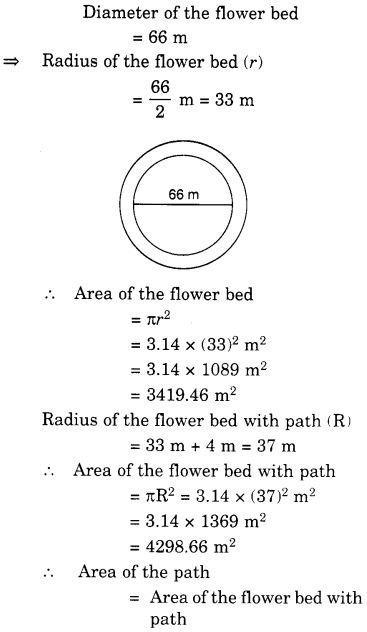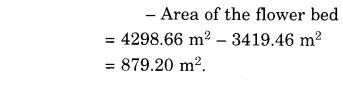Question 14.
A circular flower garden has an area of about 314 m2. A sprinkler at the centre of the garden can cover an area that has a radius of 12 m. Will the sprinkler water the entire garden? (Taken π = 3.14)
Solution:
The circular area of the sprinkler = πr2
= 3.14 × 12 × 12
= 3.14 × 144 = 452.16 m2
Area of the circular flower garden = 314 m2
Since the area of the circular flower garden is smaller than by sprinkler
Therefore, the sprinkler will water the entire garden.

Question 15.
Find the circumference of the inner and the outer circles as shown in the following figure? (Take π = 3.14)Solution:
Radius of inner circle = 19 – 10 = 9 m
∴ Circumference of the inner circle = 2 πr = 2 × 3.14 × 9 m = 56.52 cm
The radius of the outer circle = 19 m
∴ Circumference of the outer circle = 2πr = 2 × 3.14 × 19 m = 119.32 m.

Question 16.
How many times a wheel of radius 28 cm must rotate to go 352 m? ( Take π = $$\frac { 22 }{ 7 }$$ )
Solution: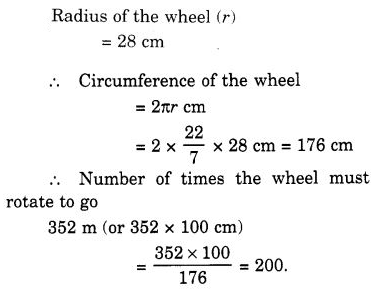Question 17.
The minute hand of a circular clock is 15 cm long. How far does the tip of the minute hand move in 1 hour? (Take π = 3.14)
Solution:
We know that the minute hand describes one complete revolution in one hour.
∴ Distance covered by its tip = Circumference of the circle of radius 15 cm
= (2 × 3.14 × 15) cm
= 94.2 cm

We hope the NCERT Solutions for Class 7 Maths Chapter 11 Perimeter and Area Ex 11.3 help you. If you have any query regarding NCERT Solutions for Class 7 Maths Chapter 11 Perimeter and Area Ex 11.3, drop a comment below and we will get back to you at the earliest.